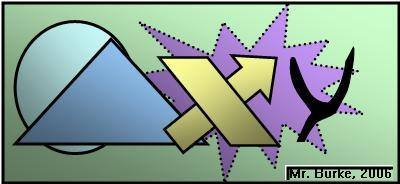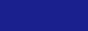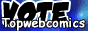Main Pageby Chris Burke1473: WeirdClick Me for a random comicSo, yes, you're weird! Weird numbers are a subset of Abundant numbers. In brief: A perfect number is one where the sum of the number's factors, excluding the number itself, equal the number. Ex: 1+2+3 = 6. An abundant number is one where the sum of the number's factors, excluding the number itself, is greater than the number. Ex: 1+2+3+4+6=16 > 12. A semiperfect number is one where a subset of the number's factors have a sum equal to the number. Ex: 1+2+3+6 = 12. A perfect number is also considered to be semiperfect, unlike my wife who is perfect and I would never consider to be semiperfect. A weird number is abundant but not semiperfect: there is no subset of factors that add up to the number. Ex: no combination of 1, 2, 5, 7, 10, 14, and 35 add up to 70, but the sum of the factors is 74. I was familiar with semiperfect, but not the "weird" term until I was looking up what the prefixes for "abundant" numbers were. Please visit my blog: http://mrburkemath.blogspot.com. (You can also go there to leave comments!)Click Me for a random comicAPR May 2019 JUN 28 29 30 1 2 3 4 5 6 7 8 9 10 11 12 13 14 15 16 17 18 19 20 21 22 23 24 25 26 27 28 29 30 31 1 2 3 4 5 6 7 8

(x, why?) is hosted on ComicGenesis, a free webhosting and site automation service for webcomics.